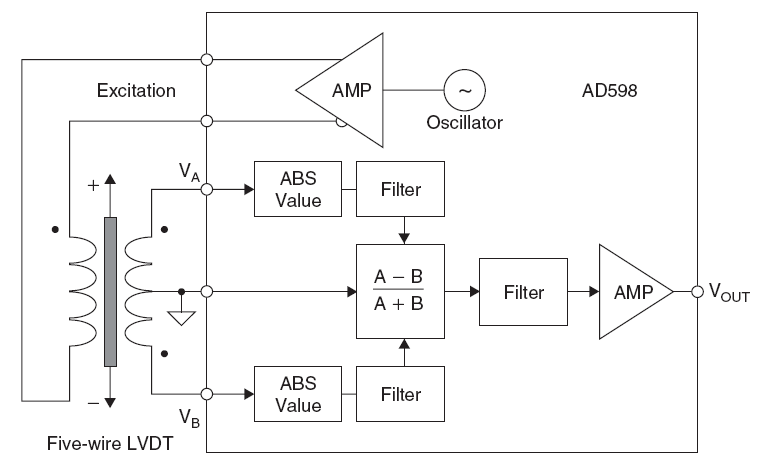# Linear Variable Differential Transformers

The linear variable differential transformer (LVDT) is an accurate and reliable method for measuring linear distance. LVDTs find uses in modern machine-tools, robotics, avionics, and computerized manufacturing.

As shown in Figure 1, the LVDT is a position-to-electrical sensor whose output is proportional to the position of a movable magnetic core. The core moves linearly inside a transformer consisting of a center primary coil and two outer secondary coils wound on a cylindrical form. The primary winding is excited with an ac voltage source (typically several kHz), inducing secondary voltages that vary with the position of the magnetic core within the assembly. The core is usually threaded in order to facilitate attachment to a non-ferromagnetic rod which, in turn, is attached to the object whose movement or displacement is being measured.Figure 1: Linear variable differential transformer (LVDT)

The secondary windings are wound out of phase with each other. When the core is centered, the voltages in the two secondary windings oppose each other, and the net output voltage is zero. When the core is moved off center, the voltage in the secondary toward which the core is moved increases, while the opposite voltage decreases. The result is a differential voltage output that varies linearly with the core’s position. Linearity is excellent over the design range of movement, typically 0.5% or better. The LVDT offers good accuracy, linearity, sensitivity, infinite resolution, frictionless operation, and mechanical ruggedness.

A wide variety of measurement ranges are available in different LVDTs, typically from ±100 μm to ±25 cm. Typical excitation voltages range from 1 V to 24 V rms, with frequencies from 50 Hz to 20 kHz. Note that a true null does not occur when the core is centered because of mismatches between the two secondary windings and leakage inductance. Also, a simple measurement of the output voltage, VOUT, will not tell the side of the null position on which the core resides.Figure 2: Improved LVDT output signal processing

A signal conditioning circuit that removes these difficulties is shown in Figure 2, where the absolute values of the two output voltages are subtracted. Using this technique, both positive and negative variations about the center position can be measured. While a diode/capacitor-type rectifier could be used as the absolute value circuit, the precision rectifier shown in Figure 3 is more accurate and linear. The input is applied to a V/I converter which, in turn, drives an analog multiplier. The sign of the differential input is detected by the comparator, whose output switches the sign of the V/I output via the analog multiplier. The final output is a precision replica of the absolute value of the input. These circuits are well-understood by IC designers, and are easy to implement on modern bipolar processes.Figure 3: Precision absolute value circuit (full wave rectifier)

The industry-standard AD598 LVDT signal conditioner, shown in simplified form in Figure 4, performs all required LVDT signal processing. The on-chip excitation frequency oscillator can be set from 20 Hz to 20 kHz with a single external capacitor. Two absolute value circuits followed by two filters are used to detect the amplitude of the A- and B channel inputs. Analog circuits are then used to generate the ratiometric function (A–B)/(A+B). Note that this function is independent of the amplitude of the primary winding excitation voltage, assuming the sum of the LVDT output voltage amplitudes remains constant over the operating range. This is the case for most LVDTs, but the user should always check with the manufacturer if it is not specified on the LVDT data sheet. Note also that this approach requires the use of a five-wire LVDT.Figure 4: AD598 LVDT signal conditioner (simplified)

A single external resistor sets the AD598 excitation voltage from approximately 1 V to 24 V rms. Drive capability is 30 mA rms. The AD598 can drive an LVDT at the end of 300 feet of cable, as the circuit is not affected by phase shifts or absolute signal magnitudes. The position output range of VOUT is ±11 V for a 6-mA load, and it can drive up to 1,000 feet of cable. The VA and VB inputs can go as low as 100 mV rms.

The AD698 LVDT signal conditioner (Figure 5) has similar specifications as the AD598, but processes the signals slightly differently using synchronous demodulation. The A and B signal processors each consist of an absolute value function and a filter. The A output is then divided by the B output to produce a final output that is ratiometric and independent of the excitation voltage amplitude. Note that the sum of the LVDT secondary voltages does not have to remain constant in the AD698.Figure 5: AD698 LVDT signal conditioner (simplified)

The AD698 can also be used with a half-bridge LVDT (similar to an auto-transformer), as shown in Figure 6. In this arrangement, the entire secondary voltage is applied to the B processor, while the center-tap voltage is applied to the A processor. The half-bridge LVDT does not produce a null voltage, and the A/B ratio represents the range-of-travel of the core.Figure 6: Half-bridge LVDT configuration

Note that the LVDT concept can be implemented in rotary form, in which case the device is called a rotary variable differential transformer (RVDT). The shaft is equivalent to the core in an LVDT, and the transformer windings are wound on the stationary part of the assembly. The RVDT is linear over a relatively narrow range of rotation, however, and is not capable of measuring a full 360° rotation. Although capable of continuous rotation, typical RVDTs are linear over a range of about ±40° about the null position (0°). Typical sensitivity is 2.5 mV/V/degree of rotation, with input voltages in the range of 3 V rms at frequencies between 400 Hz and 20 kHz. The 0° position is marked on the shaft and the body.

### Further Reading

Zumbahlen, Hank, ed. Linear Circuit Design Handbook. Newnes. 2008